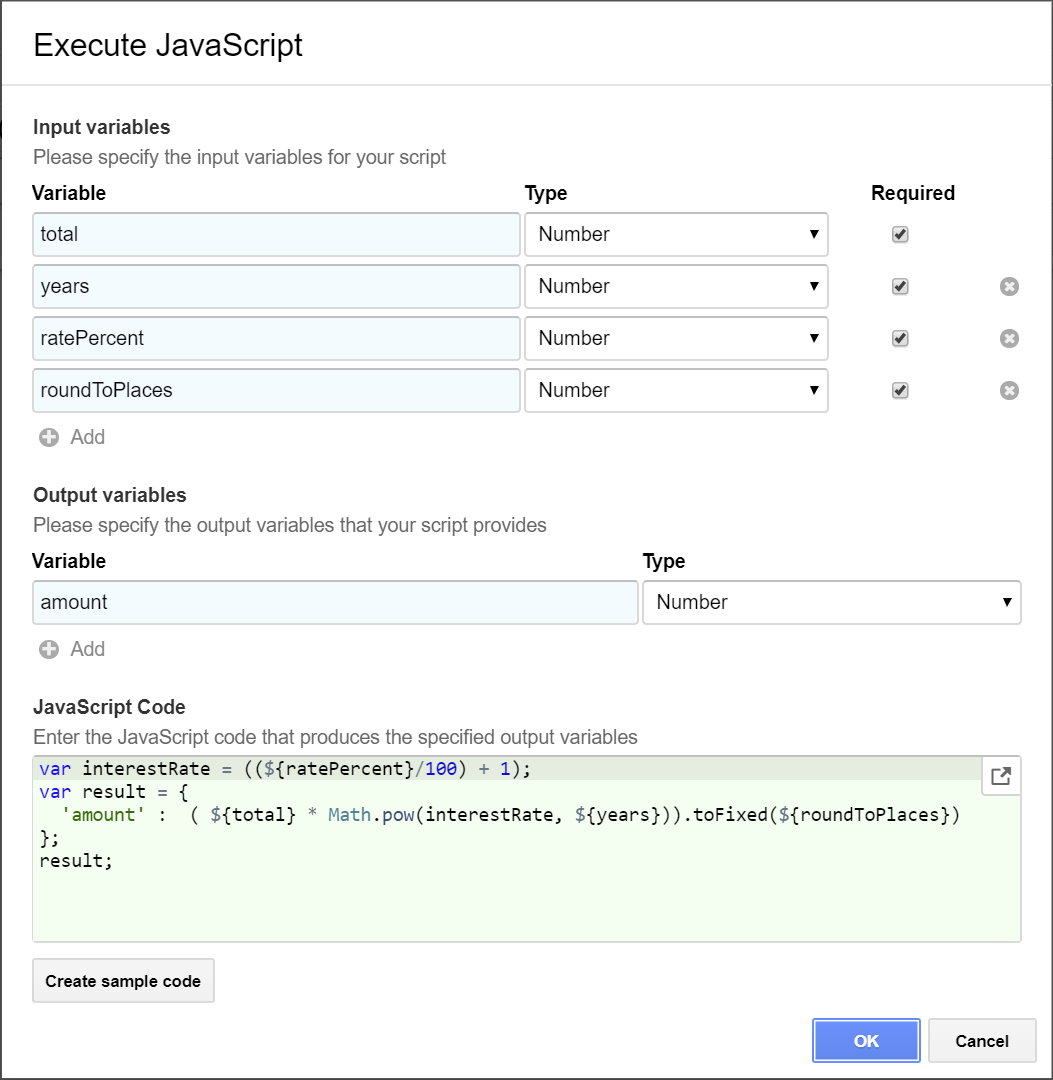JavaScript

Execute JavaScript code to perform calculations or evaluate expressions

 Execute JavaScript 2

The JavaScript block allows you run JavaScript code without the hassle of publishing Google Apps Script code.

This is perfect for all kinds of calculations, advanced conditions etc. on your existing variables.

As the script engine is baked right into Ultradox the execution is more reliable and efficient.

Use cases[a][b][c][d]

• Perform calculations

Configuration

Click on JavaScript in the building block title to open the configuration dialog.

Examples

Expressions

Sometimes you may want to use complex `if` statements in your templates. Ultradox allows you to nest if statements in your templates, but in order to keep the templates clean, the capabilities are limited.

With JavaScript you can create new variables holding the result of complex expressions and you can then use these new variables.

Calculations

Let's say you want to create a script  that returns the money you have after a given number of years of interest.

First you'll have to define the input and output variables of your script.

In this case all input variables are required, so make sure the boxes are checked.

The output variable `amount` is a number.

You can click on `Create sample code` if you want to get a starting point that takes your defined variables into account.If you are too lazy to write the code by yourself, just copy and paste the temaplte from the playground below into the script editor.

Click on `OK` to close the configuration dialog. You will then find the input and output variables in your buiding block.

In order to test you script, click on the keyboard icon in the input parameter section. This will create a matching form that you can use to assign values for the required variables.You may also want to add a log statement to double check the calculated amount by clicking on the log button in the output parameter section of your script building block.

You can then run your flow to test the script.

Playground

Before executing the script Ultradox will replace all variables with the entered values.

The calculated `amount` will be returned as part of the result and can then be used in your templates or subsequent building blocks.

You can modify the interactive sample to get an idea how Ultradox will first evaluate the template and then use the generated JavaScript code to produce the result.

```var interestRate = ((\${ratePercent}/100) + 1);
var exact = (\${total} * Math.pow(interestRate,\${years}));
// Round the exact amount to give number of places
var rounded = new Number(exact.toFixed(\${roundToPlaces}));
var result = {
'exact' : exact,
'amount' : rounded,
}
result;```
```{
"ratePercent" : "4.1",
"total" : 11250.34,
"years" : 6,
"roundToPlaces" : 2
}```

Working with complex data

With JavaScript you can also process complex data like variables containing lists.

Let's say you have a list of invoices, each of them has the fields `number` and an `amount`.

To access the list of invoices, define two input variables for your script: `invoices[].number` and `invoices[].amount`

Define the output variables that you want to generate with your script. You can return simple values as seen in the previous example, but you can also return complex data list lists.

To see this in action we will define two output variables: total and invoices[].roundedAmount

The `total` will just be a sum of all amounts, the `roundedAmount` will be added to each entry in the list to give you an idea, how to modify lists.

Generating the sample code will show you how to get the complex data into and out of your script.

Playground

Feel free to modify the template and the data to understand how Ultradox merges complex data into your code.

In the `JavaScript` tab you will find the generated code after merging the variables into the script.

```// Complex data can be passed into the script by using the object renderer
// Empty brackets will be used as default if your variable does not exist
var invoices = \${invoices([]);object(of=json)};

var total = 0;
// Loop over all invoices
for ( var i = 0; i < invoices.length; i++ ) {
// Fetch amount from each invoice...
var invoice = invoices[i]
var amount = invoice['amount'];
// ...add it to the total...
total += amount;
// ...and add new property 'roundedAmount'
invoice['roundedAmount'] = new Number(amount.toFixed(2));
}

// Round the total
total = total.toFixed(2);

// Print the rounded total to the console
console.log("Invoice total="+total);

// Return the modified list and the calculated total
var result = {
'invoices' : invoices,
'total' : total
};
result;```
```{
"invoices" : [
{
"invoice" : "NR13244-01",
"amount" : 100.25123
},
{
"invoice" : "NR13444-02",
"amount" : 199.5102
}
]
}```

Debugging

You can use `console.log()` and `console.error()` in your scripts to print log messages to the Ultradox console.

[a]Can I use javascript to perform a call to a service the same as webhook feature? I cannot use existing webhook while service has custom authentication token that should be created on a fly based on a current date.

[b]You can use JavaScript to create the token and then call the webhook using the generated token.

[c]_Marked as resolved_

[d]_Re-opened_